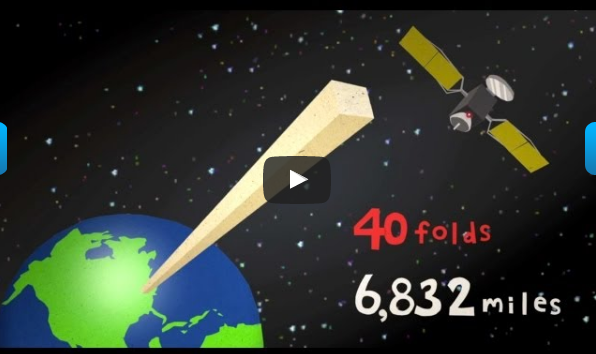# How to fold a sheet 42 times to get you to the moon? Let's try it mathematically

in science •  2 years ago

How to fold a sheet 42 times to get you to the moon? Let's try it mathematically

If it is possible to fold a sheet of paper 42 times, can you imagine how high it will be?

• 10 cm? 100 cm? No, the height of the folded paper will reach the moon!In fact, you will not normally be able to fold paper more than 7 times using your hands. However, American high school student Britney Gullivan managed to break the record by folding a piece of toilet paper 1200 meters long 12 times, and she developed an equation explaining the relationship between thickness and length of the paper and how many folds can be folded.Suppose you have a very large sheet of paper, maybe a page size of the newspaper, and now let's start with half of it

How many times can you do that fold?

Suppose you have a very large sheet of paper, maybe a page size of the newspaper, and now let's start with half of it. How many times can you do that fold?

! Unfortunately it is impossible that we flip a sheet more than seven or wish times practically

Well we did not disappoint your thinking waiting we will complete the hypothesis mathematically calculations

But to ignore this equation, imagine that we can fold a typical paper (about 0.1 mm thick) as often as we want. Let the thickness of the paper x, if the first folds will become the thickness of 2 * x and will become 4 * x the second time, and so, now the number of folds n. We can write the thickness of the paper in the form of: x * (2) ^ n. Now go to the app:

If you fold the paper, which is 0.01 cm thick once, it will double its thickness, the second time it will be 4 times thicker, the third is 8 times larger, the fourth will be 16 times, and the fifth will be 32 exponential growth. The entire day - at nine the paper thickness will exceed 500 times (512 times specifically), the twentieth time your paper will be longer than the top of Everest (10 kilometers).

In the 41st time, the fish will cross half the distance to the moon, and of course at 42 the fish will double to 439,804 km

The distance of the moon (384,000) kilometers!

We can repeat that attempt if you want to go back to Earth